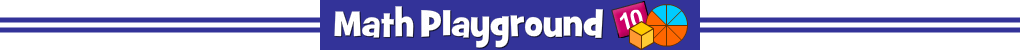Advertisement
Escape from Fraction Manner (Flash)
Escape from Fraction Manor - Learning Connections
Essential Skills
Problem Solving - complete mathematical puzzles

Common Core Connection for Grades 3 and 4
Recognize and generate simple equivalent fractions.
Explain why the fractions are equivalent by using a visual fraction model.
Compare two fractions with the same numerator or same denominator.
Compare two fractions with different numerators and different denominators.
More Math Games to Play